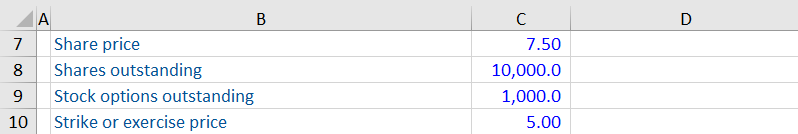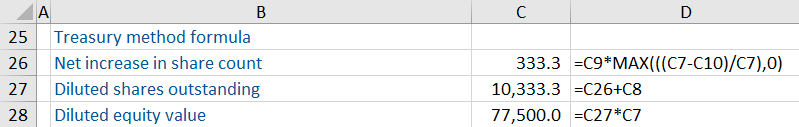## What is the Treasury Method?

The treasury method is used to calculate the number of new shares created from employee stock options and RSUs. These are contracts the company has entered into which may result in future shareholder dilution. The treasury method is therefore used to calculate the diluted number of shares and hence, the equity value or diluted earnings per share (EPS).

The calculation is completed as follows:• The treasury method assumes that the corporation will use the proceeds of the stock options exercised to buy back shares from the market and therefore minimize the dilutive impact
• MAX represents the = MAX function in Excel which returns the largest value in a set of values. This will return the largest value between net new shares created or zero

single metric. EPS is best used in conjunction with other performance indicators and, therefore, its application is limited and it should be used with care.

## Key Learning Points

• The treasury method is used to calculate the net dilution as if all stock options and RSUs contracts are exercised
• The strike (or exercise) price is the price specified within the option contract
• Dilution is only anticipated for securities that are “in the money”, if they are “under water” or “out of the money” they are not added to the share count
• The treasury method assumes that the corporation will use the proceeds of the stock option exercise to buy back shares from the market and therefore minimize the dilutive impact

## Understanding the Treasury Method

The treasury method is used to calculate the diluted number of shares. Dilution is only anticipated for securities that are “in the money” i.e. when the strike price is above the current share price.

The treasury method says that net dilution, i.e. maximum of the number of options outstanding multiplied by the share price minus the strike price, divided by the share price and zero.

Why do we do this maximum of zero or the share price minus the strike price, divided by the share price? It is because we want this to be a positive number or zero. If the share price is lower than the strike price, then the formula would return a negative number. A stock option holder will not exercise their option if the traded share price is lower than the exercise price. The options would not be exercised and hence will add 0 new shares to the count.

When calculating the net new shares for RSUs, the strike price is always 0. This is the only difference in methodology between RSUs and employee stock options. Don’t forget, another term for strike price is the exercise price.

## Dilutive Instruments

There are two dilutive instruments where the treasury method can be used to calculate the net dilution.

• Employee stock options
• Restricted share units (also known as RSUs)

The number of new shares created as a result of exercising the instruments must be added to the shares outstanding when calculating the diluted number of shares outstanding.

For employee stock options and RSUs we use the treasury method of adjustment, with a slight adjustment for the RSUs.

Convertible bonds must also be included in the diluted shares count but uses an “as if converted” method, i.e. if the convertible bonds were converted today (assuming they are in the money), how many new shares would be created.

## Treasury Method Calculation

Calculate equity value using the information below:Equity market = Share price x Diluted shares outstanding

It is the total market value of a company’s outstanding shares. The treasury stock method can be used to calculate the net dilution as a result of the stock options.

Using the formula this gives a total net share increase of 333.3. The net shares are added to the number of shares outstanding. This is then multiplied by the share price to calculate the diluted equity value of 77,500.0.The treasury method is just one method of calculating the net dilution. Download the Excel worksheet to better understand the breakdown of the calculation.